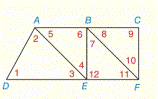Chapter 2.CR, Problem 11CR### Elementary Geometry for College St...

6th Edition
Daniel C. Alexander + 1 other
ISBN: 9781285195698

#### Solutions

Chapter
Section### Elementary Geometry for College St...

6th Edition
Daniel C. Alexander + 1 other
ISBN: 9781285195698
Textbook Problem
1 views

# For Review Exercises 7 to 11, use the given information to name the segments that must be parallel. If there are no such segments, write “none.” Assume A-B-C and D-E-F.(use the drawing from Exercise 6.) ∠ 8 ≅ ∠ 5 ≅ ∠ 3To determine

To find:

The parallel line segments.

Explanation

Given:

The given statement is,

853.

Figure (1)

Property:

(1) If two lines are cut by transversal so that two corresponding angles are congruent, then the lines are parallel.

(2) If two lines are cut by transversal so that two alternate interior angles are congruent, then the lines are parallel

Approach:

The given statement is,

853.

Lines AE¯ and BF¯ are cut by transversal AC¯ so that two corresponding angles 5 and 8 are congruent.

So,

AE¯BF¯

### Still sussing out bartleby?

Check out a sample textbook solution.

See a sample solution

#### The Solution to Your Study Problems

Bartleby provides explanations to thousands of textbook problems written by our experts, many with advanced degrees!

Get Started

#### Solve the equations in Exercises 126. x69x4=0

Finite Mathematics and Applied Calculus (MindTap Course List)

#### Evaluate the integral. 10102exsinhx+coshxdx

Single Variable Calculus: Early Transcendentals

#### True or False: f(x)=x2(x+1)x is continuous for all real numbers.

Study Guide for Stewart's Single Variable Calculus: Early Transcendentals, 8th